# 太巧妙了: 实际跑的 SVD 分解原来是这样实现的

02/02 21:00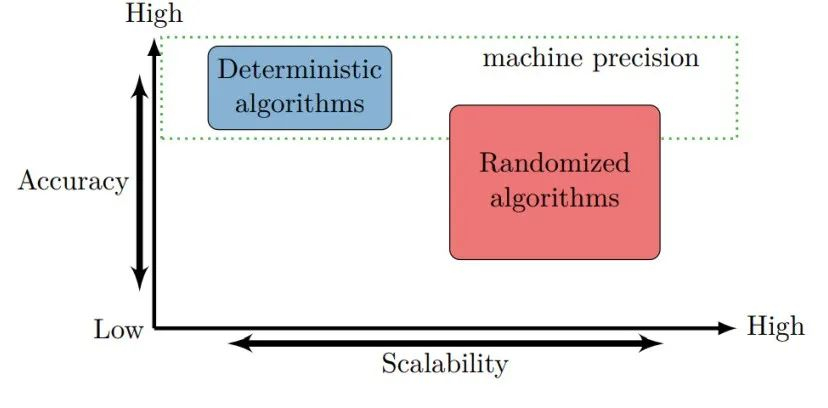## 1引言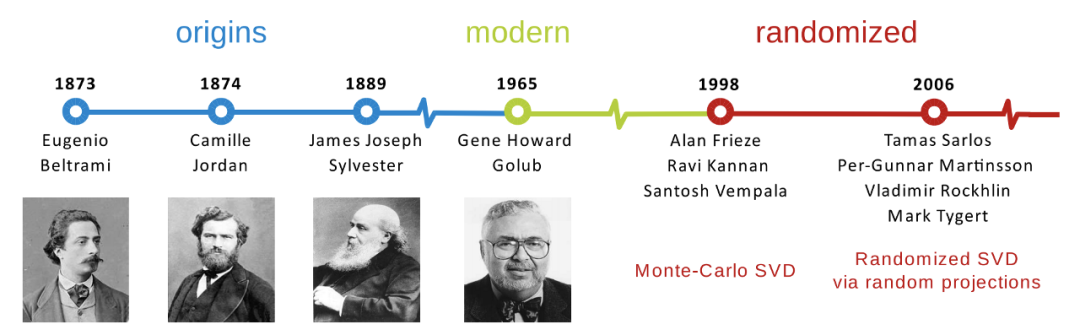SVD 技术发展时间表

## 2SVD 分解

### .小矩阵示例

import numpy as npA = np.array([[1, 3, 2],              [5, 3, 1],              [3, 4, 5]])u, s, v = np.linalg.svd(A, full_matrices = 0)print('Left singular vectors:')print(u)print()print('Singular values:')print(s)print()print('Right singular vectors:')print(v)print()
Left singular vectors:[[-0.37421754  0.28475648 -0.88253894] [-0.56470638 -0.82485997 -0.02669705] [-0.7355732   0.48838486  0.46948087]]Singular values:[9.34265841 3.24497827 1.08850813]Right singular vectors:[[-0.57847229 -0.61642675 -0.53421706] [-0.73171177  0.10269066  0.67383419] [ 0.36051032 -0.78068732  0.51045041]]

## 3随机 SVD

### .基本思想

• 0：确定目标秩
• 1：使用随机投影 采样列空间，找到一个 列矩阵 ，其列近似表示 的列空间，即
• 2：将 投影到 子空间，即 ，并在 上计算 SVD 分解。
• 3：使用 以及 的 SVD 分解得到 的左奇异向量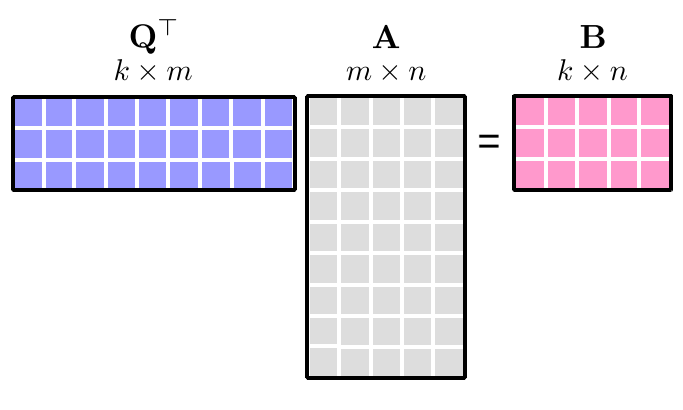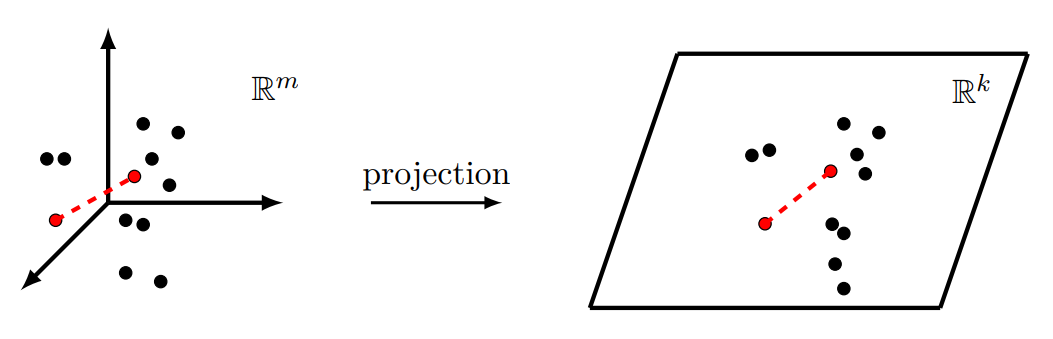### .详细步骤

• a）生成大小为 的高斯随机矩阵
• b）计算一个新的 矩阵
• c）将 QR 分解应用于矩阵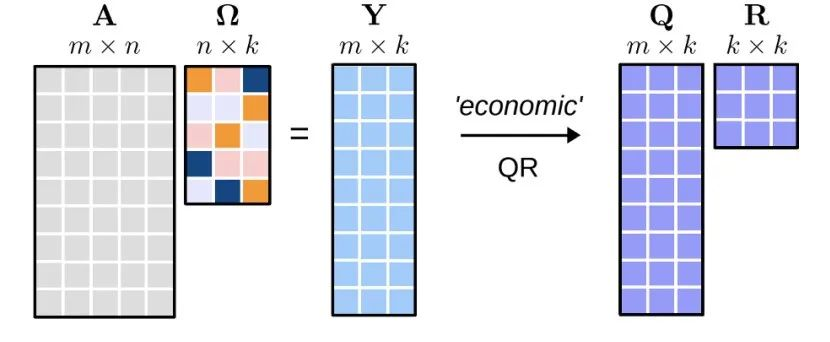• a）通过将 和矩阵 相乘得出 矩阵 ，即
• b）计算矩阵 的 SVD，用于代替计算原始矩阵 的 SVD。这里， 是一个较小的矩阵。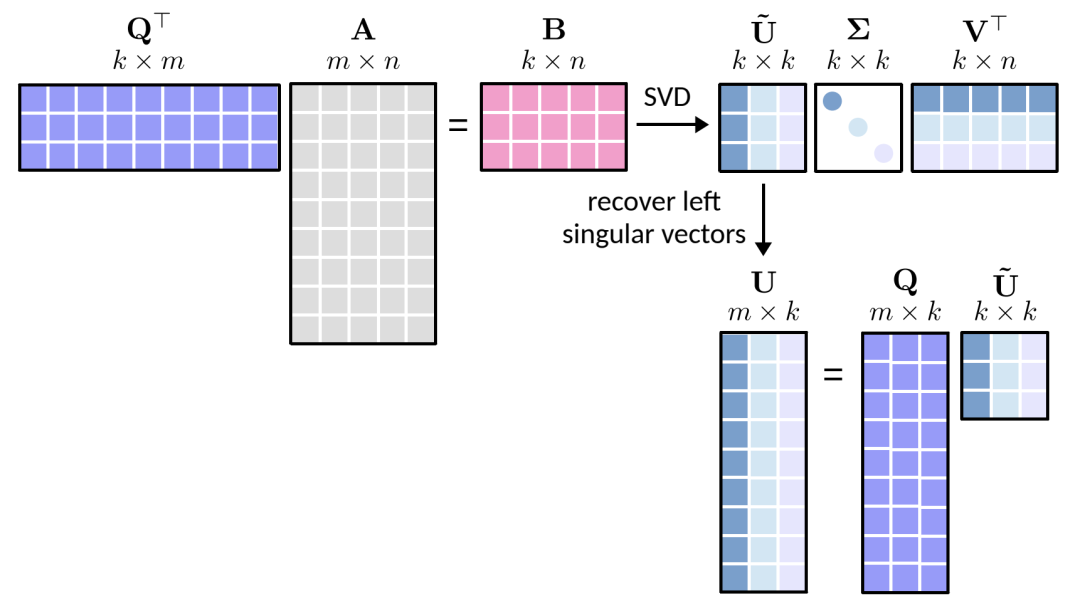### .小矩阵示例

import numpy as npdef rsvd(A, Omega):    Y = A @ Omega    Q, _ = np.linalg.qr(Y)    B = Q.T @ A    u_tilde, s, v = np.linalg.svd(B, full_matrices = 0)    u = Q @ u_tilde    return u, s, v

np.random.seed(1000)A = np.array([[1, 3, 2],              [5, 3, 1],              [3, 4, 5]])rank = 2Omega = np.random.randn(A.shape, rank)u, s, v = rsvd(A, Omega)print('Left singular vectors:')print(u)print()print('Singular values:')print(s)print()print('Right singular vectors:')print(v)print()
Left singular vectors:[[ 0.38070859  0.60505354] [ 0.56830191 -0.74963644] [ 0.72944767  0.26824507]]Singular values:[9.34224023 3.02039888]Right singular vectors:[[ 0.57915029  0.61707064  0.53273704] [-0.77420021  0.21163814  0.59650929]]

## 4幂次迭代法随机 SVD

import numpy as npdef power_iteration(A, Omega, power_iter = 3):    Y = A @ Omega    for q in range(power_iter):        Y = A @ (A.T @ Y)    Q, _ = np.linalg.qr(Y)    return Qdef rsvd(A, Omega):    Q = power_iteration(A, Omega)    B = Q.T @ A    u_tilde, s, v = np.linalg.svd(B, full_matrices = 0)    u = Q @ u_tilde    return u, s, v

np.random.seed(1000)A = np.array([[1, 3, 2],              [5, 3, 1],              [3, 4, 5]])rank = 2Omega = np.random.randn(A.shape, rank)u, s, v = rsvd(A, Omega)print('Left singular vectors:')print(u)print()print('Singular values:')print(s)print()print('Right singular vectors:')print(v)print()
Left singular vectors:[[ 0.37421757  0.28528579] [ 0.56470638 -0.82484381] [ 0.73557319  0.48810317]]Singular values:[9.34265841 3.24497775]Right singular vectors:[[ 0.57847229  0.61642675  0.53421706] [-0.73178429  0.10284774  0.67373147]]

### 参考资料



Randomized Matrix Decompositions Using R.: https://arxiv.org/pdf/1608.02148.pdf



Randomized Singular Value Decomposition: http://gregorygundersen.com/blog/2019/01/17/randomized-svd/



# 矩阵特征值是这么来的，以及有趣的盖尔圆

## 矩阵之芯 SVD: 基本应用以及与其他分解的关系0
0 收藏

### 作者的其它热门文章0 评论
0 收藏
0